## Time step in FlowVision. How to specify - Why are there so many criteria?

### Why are there so many criteria and explicit steps?

FlowVision simulates various processes for which it is necessary to solve different equations: equation of fluid motion, multiphase interaction, heat transfer, mass transfer and many others. One or another criterion for calculating the time step is selected depending on the problem to be solved, .

Let's consider a boat floating along the waves. The following processes are modeled to obtain a solution of such task:

• Water motion;
• Boat movement;
• Free surface motion - phase boundary: water - air.

Diffusion proccesses and sliding surface are not taken into account, that means there is no point in considering these criteria for calculating the final time step for this particular task. The convective and surface criteria are used.

It is necessary to specify surface CFL = 1 for tasks with moving bodies.

Or another example: the problem of particles mass transfer. The processes, that are modelled in this task:

• Phase motion;
• Motion of dispersed particles.

There is still no sliding surface, also there is no moving bodies and free surface movement. Therefore, only convective and diffusion criteria will be used.

If you have a rotation with sliding surfaces it is neccessary to spesify slide CFL = 1

If a physical process that defines the criterion is not considered in your task, then the criterion (CFL number) should be assigned the value 1e + 20. In such a setting, the criterion will not be taken into account.
Let's have a look at each of the criteria and the corresponding explisit time step.

#### convective CFL

Is used in the tasks, where motion is simulated.

General recommendation for choosing a value for this criterion is to perform an investigation on this time step.

All tasks are different and require own specific time step. Therefore, it is necessary to investigate the dependence of the characteristic control value on the time step.
For example, examining the pressure drop in a pipe with a variable velocity at the inlet, it can be noted how the value of the pressure drop is changing at different steps. At a certain value of the time step (t <20), the control value doesn't change - this is the required time step.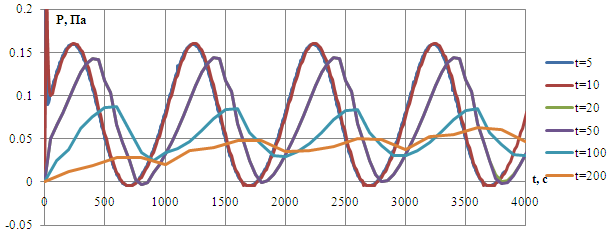The time step is calculated as follows: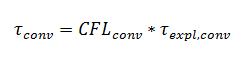Convective CFL scales convective time step, which is calculated by formula: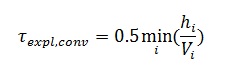To calculate the explicit time step, FlowVision determines the minimum ratio of a cell size to velocity in that cell within the entire subregion. If you know the size of a cell and can estimate a velocity value for a specific task, you can also calculate the step in volume by the formula.

For supersonic flows, it is recommended to use convective CFL 1 ... 10, for subsonic flows it is possible to start with higher values 30…100 CFL.

#### Diffusive CFL

Is used for diffusive time step calculation: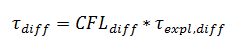It means, the diffusive CFL also scales explicit diffusive time step: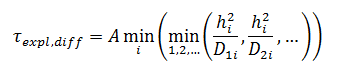where A = 1 is set for implicit scheme and A = 0.25 - for explicit scheme, ensuring its stability.

The explicit diffusive step is calculated as the minimum time during which the velocity perturbation propagates diffusionally in the cell. This step is used for viscous flows simulations, chemical reactions and anisotropy.

It is recommended to set up diffusive CFL from 10 to 1000.

#### surface CFL

It is used in any tasks where the motion of a free surface or moving bodies is simulated. This criterion limits the step so that the body or surface cannot cross more than one cell in one iteration. If the body will cross more than one cell at a time, then the calculation will turn out to be far from physical.

The step size for such tasks is appropriate: surface CFL= 1.

It is calculated as: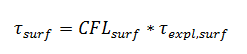where the explicit step is calculated by FlowVision as minimum of the ratio of the size of a cell containing a free surface or a moving body to the velocity modulus at the phase/moving body boundary: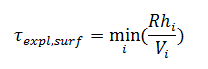R –coefficient that increases the stability of calculation. For a free surface, R = 0.5, and for a moving body, R = 1.

#### Slide CFL

Calculates the time step for a sliding surface: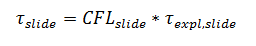Explicit time step is calculated as the minimum ratio of the cell size on a sliding surface to the linear velocity on this sliding surface.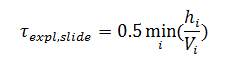It is specified strictly as 1.

If a "frozen rotor" is used - it is not taken into account in time step calculation.